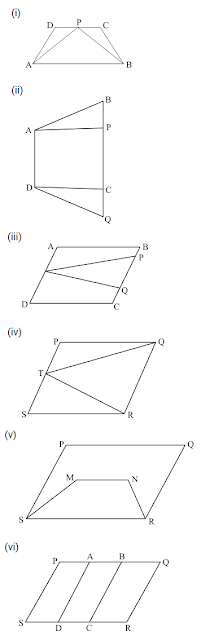#### Chapter 15 Areas of Parallelogram and Triangles R.D. Sharma Solutions for Class 9th Exercise 15.1

Exercise 15.1

1.Which of the following figures lie on the same base and between the same parallels. In such a case, write the common base and two parallels:Solution

(i) ΔPCB and trapezium ABCD are on the same base CD and between the same parallels AB and DC.

(ii) Parallelograms ABCD and APQD are on the same base AD and between the same parallels AD and BQ.

(iii) Parallelogram ABCD and ΔPQR are between the same parallels AD and BC but they are not on the same base.

(iv) ΔQRT and parallelogram PQRS are on the same base QR and between the same parallels QR and PS.

(v) Parallelograms PQRS and trapezium SMNR are on the same base but not between the same parallels.

(vi) Parallelograms PQRS, AQRD, BCQR are between the same parallels. Also, parallelograms PQRS, BPSC, APSD are between the same parallels.

(vi) Parallelograms PQRS, AQRD, BCQR are between the same parallels. Also, parallelograms PQRS, BPSC, APSD are between the same parallels.